Payal Tandon
Co-founder, e-GMAT
Welcome to e-GMAT Support!
I am Payal, Co-Founder of e-GMAT.
Feel free to ask any Query.We will be contacting you soon on# Difference between Permutation and Combination explained with examples

Permutations and Combinations is a topic full of conundrums. The biggest one is, understanding the difference between permutation and combination. Should I solve this...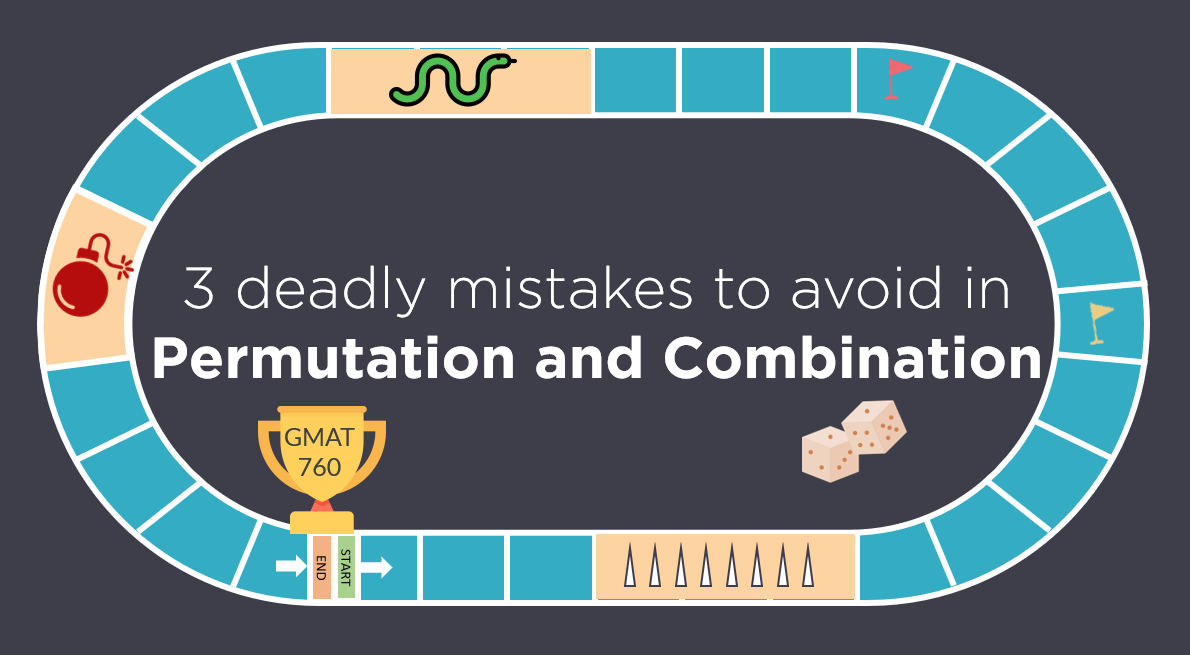# Permutation and Combination | Avoid these 3 Mistakes | GMAT Quant

Like any GMAT Quant topic, Permutation and Combination has its own traps. Most students fall in these traps and ultimately, are not able to secure their target GMAT score....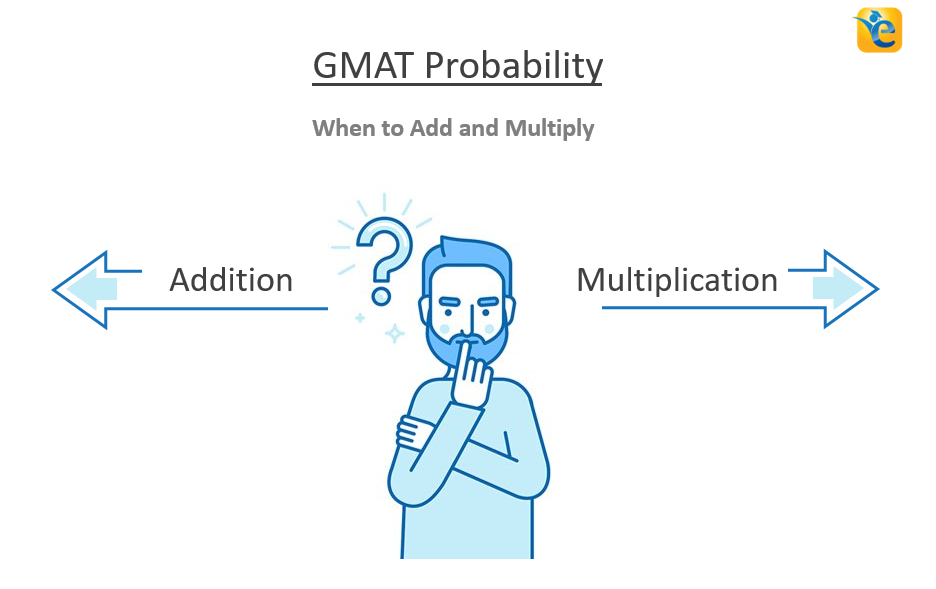# GMAT Permutation and Combination | When to Add and Multiply

Are you always confused whether to add or multiply the cases while solving GMAT permutation and combination questions? Do you often get questions incorrect simply because...

# If p, r, and s are consecutive integers in ascending order and x is the average (arithmetic mean) of the three integers – OG 2020 Question #304 with Solution

OG 2020: Question No. 304 If p, r, and s are consecutive integers in ascending order and x is the average (arithmetic mean) of the three integers,...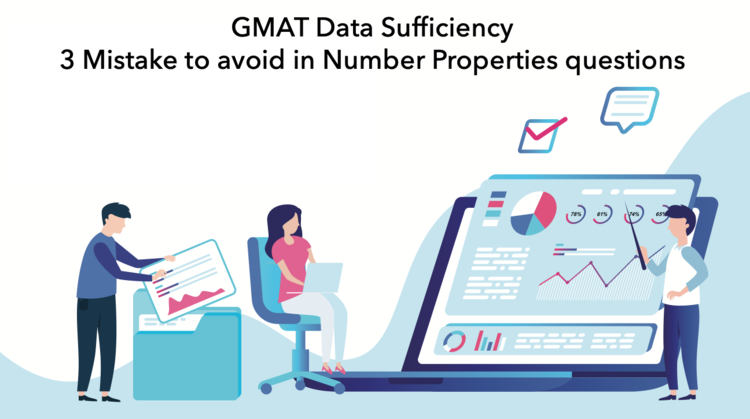# GMAT Data Sufficiency – 3 Mistake Students make in Number Properties questions

Typical GMAT data sufficiency questions are followed by information in the form of two or three statements. You have to figure out which statements...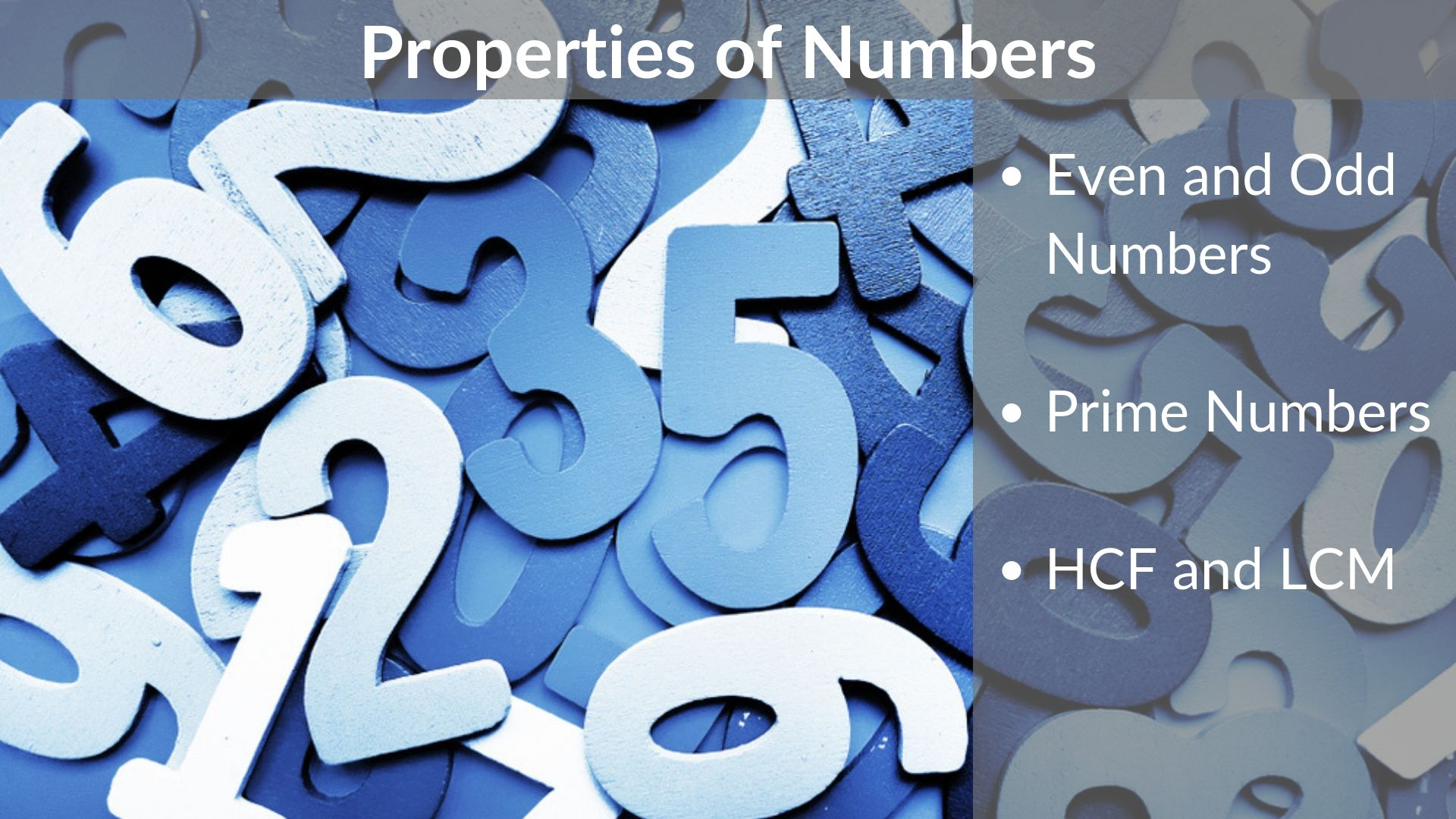# Properties of Numbers – Even & Odd | Prime | HCF & LCM

Hello Reader, We see that you want to know the basics and properties of numbers. You have landed just at the right place.  Purpose of the...# GMAT Factorials – Variations in Factorial Manipulation

On the GMAT, Factorial is an interesting concept in number properties from which many application-oriented questions are asked. In this article, we...

# Eight light bulbs numbered 1 through 8 are arranged in a circle as shown above. The bulbs are wired so that every third bulb, counting in a clockwise direction, flashes until all bulbs have flashed once. If the bulb numbered 1 flashes first, which numbered bulb will flash last? – OGQR 2020 Question #102 with Solution

OGQR 2020: Question No. 102 Eight light bulbs numbered 1 through 8 are arranged in a circle as shown above. The bulbs are wired so that every third...

# More articles

## If p, r, and s are consecutive integers in ascending order and x is the average (arithmetic mean) of the three integers – OG 2020 Question #304 with Solution

A 2 min read OG 2020: Question No. 304 If p, r, and s are consecutive integers in ascending order and x is the average (arithmetic mean) of the three integers, what is the value of x? Twice x is equal to the sum of p, r, and...

## GMAT Data Sufficiency – 3 Mistake Students make in Number Properties questions

A 7 min read Typical GMAT data sufficiency questions are followed by information in the form of...

## Properties of Numbers – Even & Odd | Prime | HCF & LCM

A 8 min read Hello Reader, We see that you want to know the basics and properties of numbers. You...

## GMAT Factorials – Variations in Factorial Manipulation

A 7 min read On the GMAT, Factorial is an interesting concept in number properties from which many...

## Eight light bulbs numbered 1 through 8 are arranged in a circle as shown above. The bulbs are wired so that every third bulb, counting in a clockwise direction, flashes until all bulbs have flashed once. If the bulb numbered 1 flashes first, which numbered bulb will flash last? – OGQR 2020 Question #102 with Solution

A 2 min read OGQR 2020: Question No. 102 Eight light bulbs numbered 1 through 8 are arranged in a circle as shown above. The bulbs are wired so that every third bulb, counting in a clockwise direction, flashes until all bulbs...

## GMAT Quant OGQR 2020 Question #86 with Solution – “If the smaller of 2 consecutive odd integers…”

A 2 min read PQID = PS91602.01  | OGQR 2020: Question No. 86 If the smaller of 2 consecutive odd integers is a multiple of 5, which of the following could NOT be the sum of these 2 integers? SourceOGQR 2020TypeProblem...

## Is the average (arithmetic mean) of the numbers x, y, and z greater than z? – OG 2020 Question #320 with Solution

A 2 min read OG 2020: Question No. 320 Is the average (arithmetic mean) of the numbers x, y, and z greater than z? z − x < y − z x < z < y Source OG 2020 Type Data Sufficiency Topic Algebra, Number Properties Sub-Topic...

## GMAT Quant OG 2020 Question #318 with Solution – “If x is an integer greater than 0…”

A 2 min read PQID = DS49502.01 | OG 2020: Question No. 318 If x is an integer greater than 0, what is the remainder when x is divided by 4? The remainder is 3 when x + 1 is divided by 4.The remainder is 0 when 2x is divided by...

## GMAT Quant OG 2020 Question No. 370 with Solution-“If 2.00X and 3.00Y are 2 numbers in decimal form with thousandths digits…”

A 2 min read PQID = DS13841.01 | OG 2020 Question No. 370 If 2.00X and 3.00Y are 2 numbers in decimal form with thousandths digits X and Y, is 3(2.00X) > 2(3.00Y)? 3X < 2YX < Y – 3 SourceOG 2020PQIDDS13841.01TypeData...

## GMAT Quant OG 2020 Question #377 with Solution – A certain list, L, contains… PQID: DS08091.01

A 2 min read PQID: DS08091.01  GMAT Quant OG 2020 Solution: Question No. 377 A certain list, L, contains a total of n numbers, not necessarily distinct, that are arranged in increasing order. If L1 is the list consisting of...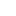###### Don't limit yourself!# Achieve 740+on the GMAT in 30 days!Sign up for our free trial and get:400+ Practice questions with detailed solutions10+ hours of AI-driven video lessonsAdaptive mock test with ESR+ analysis